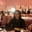Related Tags

javascript
communitycreator

# How to convert a subset of a string into an array using JSAdodoola Omiyale

### Overview

To convert a subset of a string into an array, we’ll create a function to get the subsets and return them into an empty array.

We’ll perform three steps:

1. Create a string and an empty array variable.

2. Split the string into separate arrays.

3. Create a function to get the subsets and input them into one array.

### Example

In this example, we’ll perform the first step, creating the string and array

let str ="cat"
let result=[]
console.log(str, result)
Creating the str string and result array

### Explanation

In line 2 and 3, we create a string variable with a value of cat and an empty array ([]) to store the subsets.

### Example

In this example, we convert the string into arrays using the split() and slice() methods.

let str ="cat";
str.split("").forEach(function(element, index){
console.log([...str.slice(index)])
})
Using the split method to parse the string into arrays

### Explanation

In line 2 and 3, we use the split() method to separate the characters and return the result as an array. For example [c,a,t].

We use the forEach() method to repeat a function for each array element.

In the code above, for each of the three array elements, we use the slice() method to remove the first (index) element from the first array and repeat the process for all three.

Finally, we use console.log()to display the result.

### Example

In this example, we will create the subString function to retrieve the subsets from each array shown above.

function getSubset(inputArray) {
let temp =""
inputArray.forEach (function (element,index) {
temp= temp+element ;
result.push(temp)})
}

Using the subString function to retrive the subset from each array

### Explanation

• In line 1, we create the getSubset() function to get all the subsets from the array passed as an inputArr parameter.
• In line 2, we will create an empty string variable called temp to store the subsets.
• In line 3 and 4, we use the forEach () method. For each array element, the temp variable is added. As every element is added, the temp variable is updated to create a new subset.
• In line 5, we use the push() method to add each updated subset (temp) to the end of the result array.
• This process will be repeated for all three of the arrays.
• In line 7, we use the console.log() to display the subset of all the array elements in the result array.

### Example

In this example, we will show how the getSubset function is used for one of the arrays.

function getSubset([d,o,g]) {
let temp =""
[d,o,g].forEach (function (element,index) {
temp= temp+element ;
//(1) "" = "" + d  //return = (temp=d)
//(2) d = d + o    // return = (temp=do)
//(3) do =do + g   // return = (temp = dog )
result.push(temp)})
//(1) result = [d]
//(2) result = [d, do]
//(3) result = [d, do, dog]
}


Using the getSubset function in practice

### Final code

Now, let's show all the codes above executed together.

//(1)
let str ="cat"
let result = []

//(3)
function getSubstring(inputArr){
let temp = "";
inputArr.forEach(function(element, index){
temp= temp + element;
result.push(temp);}
)}

//(2)
str.split('').forEach(function(element, index)
{getSubstring ([...str.slice(index)])
})

console.log(result)
Combining our previous steps

RELATED TAGS

javascript
communitycreator

CONTRIBUTORAdodoola Omiyale
RELATED COURSES

View all Courses

Keep Exploring

Learn in-demand tech skills in half the time